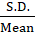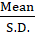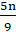Daily Practice Problems
Class 11 Maths
StatisticsQuestion 1:

Find the mean deviation about the mean for the following data:

6, 7, 10, 12, 13, 4, 8, 12

Question 2:

The variance of the given data 2, 4, 5, 6, 8, 17 is 23.33. Find the variance for the data 4, 8, 10, 12, 16, 34.

Question 3:

Find the variance and the standard deviation for the following data: 57, 64, 43, 67, 49, 59, 44, 47, 61, 59.

Question 4:

In a test with a maximum marks 25, eleven students scored 3, 9, 5, 3, 12, 10, 17, 4, 7, 19, 21 marks respectively. Calculate the range.

Question 5:

Coefficient of variation of two distributions is 70 and 75, and their standard deviations are 28 and 27 respectively what are their arithmetic mean?

Question 6:

The mean of 2, 7, 4, 6, 8 and p is 7. Find the mean deviation about the median of these observations.

Question 7:

Find the mean and variance for the first n natural numbers.

Question 8:

The sum of 10 items is 12 and the sum of their squares is 18. Find the standard deviation.

Question 9:

The algebraic sum of the deviation of 20 observations measured from 30 is 2. So, the mean of observations is _________.

Question 10:

The coefficient of variation is computed by

(a)* 100

(b)(c)* 100

(d)Question 11:

If mode of a series exceeds its mean by 12, then mode exceeds the median by

(a) 4

(b) 8

(c) 6

(d) 12

Question 12:

The median and SD of a distributed are 20 and 4 respectively. If each item is increased by 2, the new median and SD are ________.

Question 13:

If the mean of first n natural numbers is, then n = _____.

Question 14:

Find the mean deviation about the median for the data.

 xi 15 21 27 30 35 yi 3 5 6 7 8

Question 15:

The mean of a group of 100 observations was found to be 20. Later on, it was found that three observations were incorrect, which was recorded as 21, 21 and 18. Then the mean if the incorrect observations are omitted is _____.

Question 16:

Variance is independent of change of

(a) origin only

(b) scale only

(c) origin and scale both

(d) None of these

Question 17:

The mean of 5 observations is 4.4 and their variance is 8.24. If three of the observations are 1, 2 and 6, find the other two observations.

Question 18:

The mean of numbers 1, 3, 4, 5, 7, 4 is m and the numbers 3, 2, 2, 4, 3, 3, p have mean m – 1 and median q. Find the value of p + q - m.

Question 19:

The measure of dispersion is

(a) mean deviation

(b) standard deviation

(c) quartile deviation

(d) all a, b and c

Question 20:

Consider the following statements:

i. Mode can be computed from histogram.

ii. Median is not independent of change of scale.

iii. Variance is independent of change of origin and scale.

Which of these is/are correct?

(a) i, ii and iii

(b) only ii

(c) only i and ii

(d) only i

**********